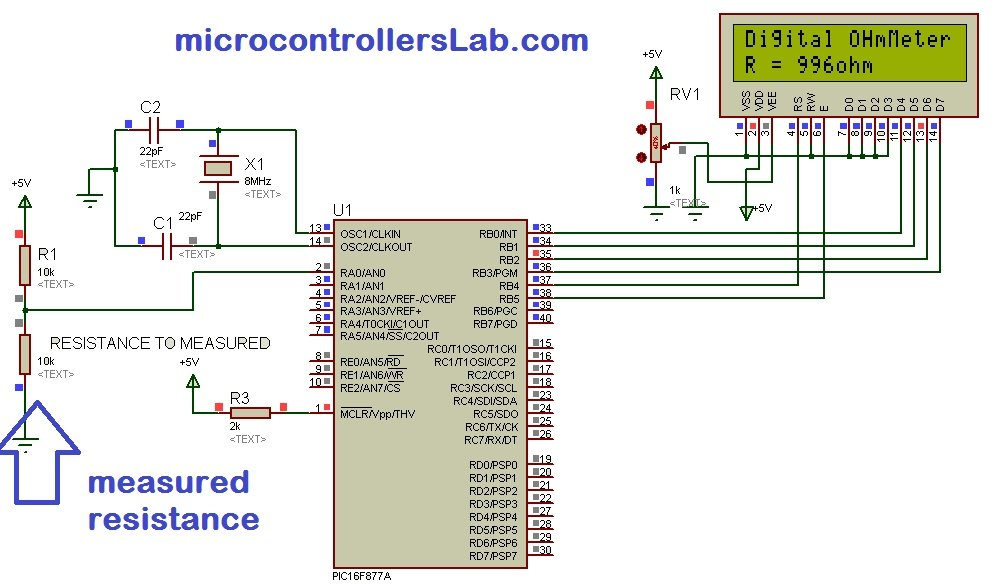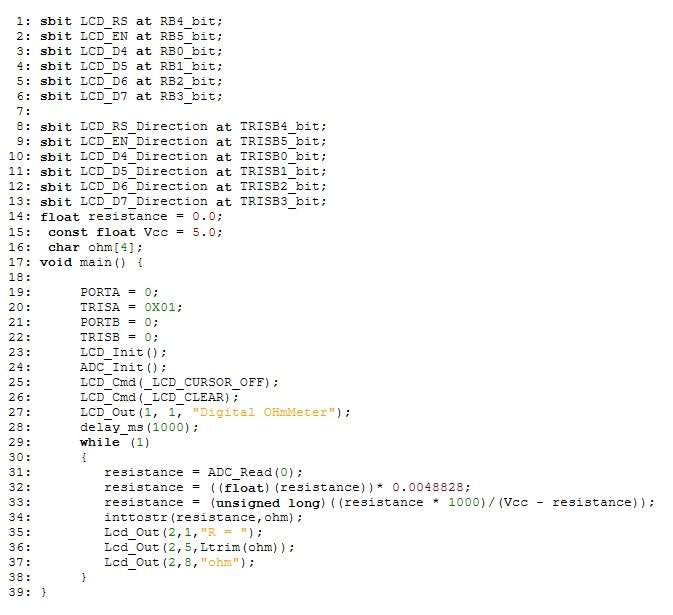# Digital Ohmmeter circuit using pic microcontroller

### Digital Ohmmeter project using pic microcontroller

Digial ohmmeter using pic microcontroller is designed in this project. Digital ohmmeter is used to measure resistance of any resistance or any circuit. It can measure resistance between 0-1MΩ with precision of ±5  percent.  PIC16F877A microcontroller is used in this project to measure resistance by doing signal conditioning of measured resistance. LCD is used to display resistance value. Voltage divider concept is also in this project. I will discuss it in later part of this article.

### Analog and digital ohmmeter

Analog ohm meter use electronics concepts to measure resistance and displays it on analog meters with the help of needles. Digital ohmmeter use numerical display to display resistance value like seven segment display. liquid crystal display and LED displays. Digital ohmmeter also use digital electronics to measure resistance with the help of microcomputers.

### digital ohmmeter Circuit diagram  using pic microcontroller

Circuit diagram of digital ohmmeter using pic microcontroller is given below. As shown in figure below, resistance R1 is pulled with 5 volt source and resistance whose value we want to measure is connect with ground and other terminal of resistance R1. Voltage divider concept is used to measure resistance value. LCD is used to display resistance value.### Digital ohmmeter working and operation

In above circuit diagram resistance R1 is used to as pull up resistor. Resistance R1 is connected to 5 volt source. The resistance whose value we want to measure is connected with resistance R1 to form a voltage divider. I have already discussed in Digital voltmeter article about how to measure voltage using pic microcontroller. I also used voltage divider in digital voltmeter article to design digital voltmeter. Same concept is used in this article. you should also know about LCD interfacing with pic microcontroller. If you don’t know about voltage measurement and lcd interfacing, I recommend you to read following articles first:

Voltage across measured resistance is measured with the help of PIC16F877A microcontroller. Voltage across measured resistance value is measured with channel ANO of PIC16F877A microcontroller.Now the question is how resistance can be determined by using measured value of voltage? It is very easy to calculate using voltage division formula. Voltage divider formula is given below:

voltageoutput = (measured resistance/ (measured resistance  +  R1)) * Vcc

Value of Vcc and resistance R1 is known which is Vcc = 5 volt and R1 = 10K ohm. So PIC16F877A microcontroller calculated voltageoutput according to connected measured resistance value. After that measured resistance value can be easily calculated by using below given formula:

Measured resistance = R1 × voltageoutput / 5 – voltageoutput;

Measured resistance = 10000 × voltageouput / 5 – voltageoutput;

Above formula is just rearranged form of voltage division formula. So above resistance can be easily calculated by using above calculations in programming. I will discuss it in programming part of digital ohmmeter project. This digital ohmmeter can measure resistance between 0-1M ohm with precision of ±5 percent.

### Digital ohmmeter program

Code for digital ohmmeter using pic microcontroller is written in Mikro C pro compiler. Step by step description of code is also given below:• sbit LCD_X at RBX_bit: It is used to define PORT B connections with LCD
• sbit LCD_X_Direction at TRISBX_bit: It is used to define direction of PORTB as input and output
• LCD_Init(): This function initialized the LCD functions.
• resistance = ((float)(resistance))* 0.0048828 : It converts ADC binary value back into voltage output value.
•  resistance = (unsigned long)((resistance * 1000)/(Vcc – resistance)) : This statement converts output voltage of ADC into measured resistance value by using voltage divider formula
•   inttostr(resistance,ohm) : It converts resistance value into string to display it on LCD
• Rest all the instructions in code is self-explanatory. But if you still feel any problem, your comments are welcome.

You may also like to read:

### 10 thoughts on “Digital Ohmmeter circuit using pic microcontroller”

1. Good day sir. There’s some error I’ve experienced in coding in the line resistance = ADC_Read(0): It says error “assigning to non-lvalue ‘resistance’ What should I do with it?

2. Good day sir, Thank you for sharing this digital ohmmeter circuit with us. I try to understand the program.
There’s something thing i wanna ask you about the formula that you use.
The resistance coding
resistance = ((float)(resistance))* 0.0048828;
resistance = (unsigned long)((resistance * 1000)/(Vcc – resistance));
1. How you get the numbers of 0.0048828?
2.How you manipulated the voltage divider formula to write formula in programming?

Thank you sir,

• have you replaied any answer ??!

• 0.0048828 is from ADC -> where you have: ADC(value ) * 5 / 1023, and 5/1023 = 0.0048828

3. Digital Ohmmeter
Good day sir,
Can you send me by e-mail the file “.HEX ”
Thank you
Jimmy

4. Its 5/1024=0.000488
5v from vref+ and 1024 possible values for de A/d converter (10bit converter 2^10)

5. Hi,
resistance = (unsigned long)((resistance * 1000)/(Vcc – resistance)) :
I think this equation is wrong, instead of 1000 use 10000.